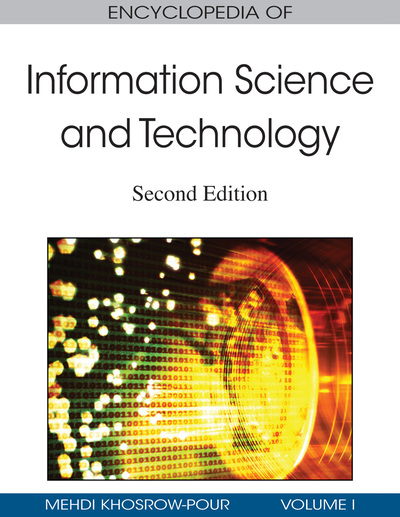# Ant Colony Algorithms for Data Classification

Alex A. Freitas (University of Kent, UK), Rafael S. Parpinelli (UDESC, Brazil), and Heitor S. Lopes (UTFPR, Brazil)
DOI: 10.4018/978-1-60566-026-4.ch027
Available
\$29.50
No Current Special Offers

Chapter Preview
Top

## Background

An ACO algorithm is essentially a computational system inspired by the behavior of natural ants and designed to solve real-world optimization problems. The basic ideas of ACO algorithms are as follows (Dorigo & Stutzle, 2004):

• Each ant incrementally constructs a candidate solution to a given optimization problem. That candidate solution is associated with a path in a graph representing the search space.

• When an ant follows a path, the amount of pheromone deposited on that path is proportional to the quality of the corresponding candidate solution.

• At each step during the incremental construction of a candidate solution, an ant typically has to choose which solution component should be added to the current partial solution (i.e., how to extend the current path), among several solution components. In general the probability of a given component being chosen is proportional to the product of two terms: (a) the amount of pheromone associated with that component; and (b) the value of a (problem-dependent) heuristic function for that component.

As a result, due to a continuous increase of the pheromone associated with the components of the best candidate solutions considered by the algorithm, the ants usually converge to the optimum or near-optimum solution for the target problem.

The motivation for applying ACO algorithms to the discovery of classification rules and related data mining tasks is as follows. Many projects in the field of data mining proposed deterministic rule induction algorithms. These algorithms typically are greedy, and so they are susceptible to find only locally optimal (rather than globally optimal) classification rules. By contrast, ACO algorithms try to mitigate this drawback using a combination of two basic ideas. First, these algorithms have a stochastic aspect, which helps them to explore a larger area of the search space. Second, they use an iterative adaptation procedure based on positive feedback (the gradual increase of the pheromone associated with the best solution components) to continuously improve candidate rules. Combining these basic ideas, in general ACO algorithms perform a more global search in the space of candidate rules than typical deterministic rule induction algorithms, which makes the former an interesting alternative to be considered in rule induction.

The first ACO algorithm proposed for discovering classification rules was Ant-Miner (Parpinelli et al., 2002a). In Ant-Miner each artificial path constructed by an ant represents a candidate classification rule of the form:

• IF <term1 AND term2 AND … > THEN < class >.

## Key Terms in this Chapter

Test: Set: A set of cases unseen during the training of the algorithm. The test set is used to measure the predictive accuracy (generalization ability) of the rules discovered during training.

Data Mining: A research field where the goal is to discover accurate, comprehensible knowledge (or patterns) in data.

## Complete Chapter List

Search this Book:
Reset